Hot Posts:

# How to combine multiple sheet(Worksheet) into one sheet

• 0
• 6839© How to combine multiple sheet(Worksheet) into one sheet
Font size:
Print

This tutorial shows you how to combine or merge multiple excel sheets (worksheets) into one in just 1 minutes. its lot easier to share or make operations on single sheet. so Just follow these steps.

1. I have a excel file that contain 4 sheets that has different data but common title row. As shown in image below.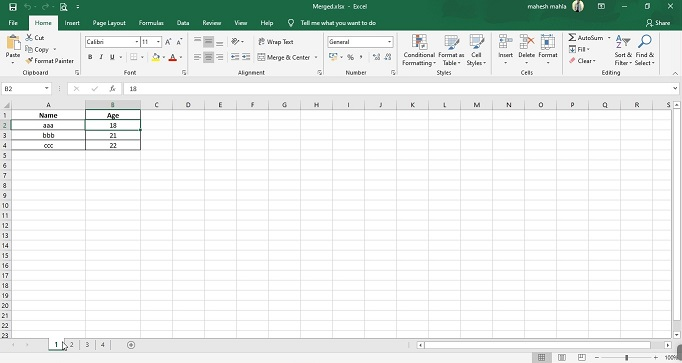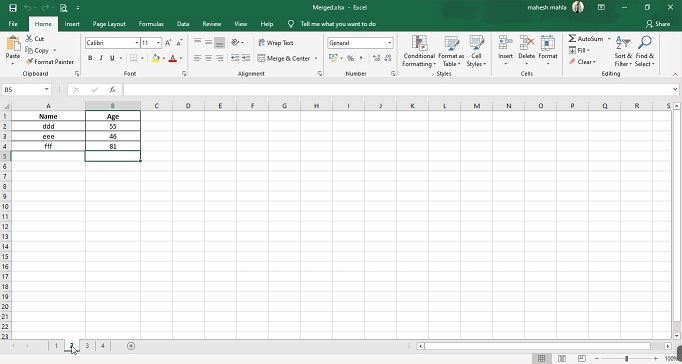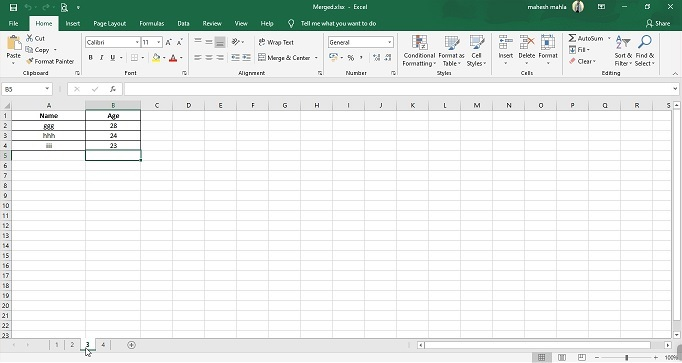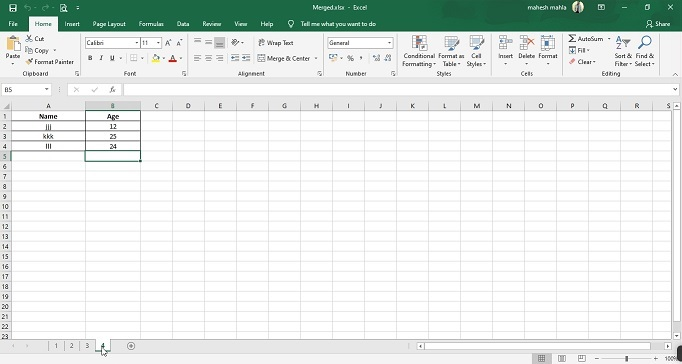2. Now open VBA editor by pressing keys Alt+F11 and then open module in insert menu.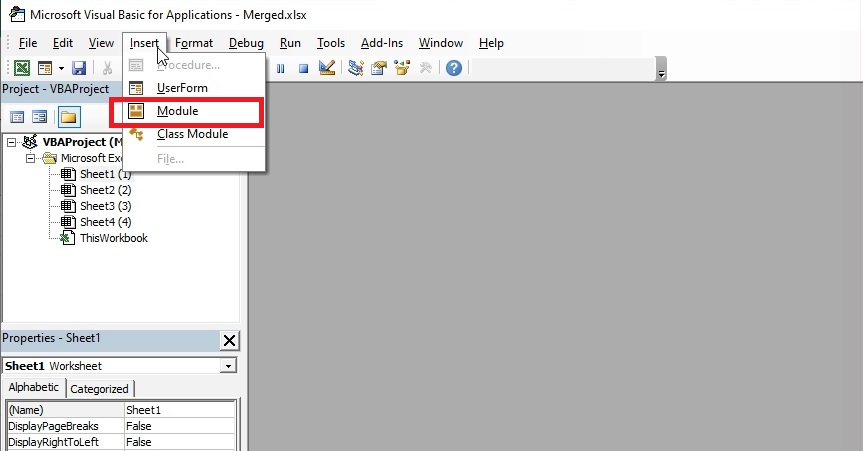3. Now copy and paste below given code in this editor.

Sub Combine()

'UpdateByKutools20151029

Dim i As Integer

Dim xTCount As Variant

Dim xWs As Worksheet

On Error Resume Next

LInput:

xTCount = Application.InputBox("The number of title rows", "", "1")

If TypeName(xTCount) = "Boolean" Then Exit Sub

If Not IsNumeric(xTCount) Then

MsgBox "Only can enter number", , "Mahesh Mahala"

GoTo LInput

End If

xWs.Name = "Combined"

Worksheets(2).Range("A1").EntireRow.Copy Destination:=xWs.Range("A1")

For i = 2 To Worksheets.Count

Worksheets(i).Range("A1").CurrentRegion.Offset(CInt(xTCount), 0).Copy _

Destination:=xWs.Cells(xWs.UsedRange.Cells(xWs.UsedRange.Count).Row + 1, 1)

Next

End Sub

4. Press Run button to run code it will ask for title rows. here in these sheets title row is 1. you enter as per your sheet's row. And hit OK button.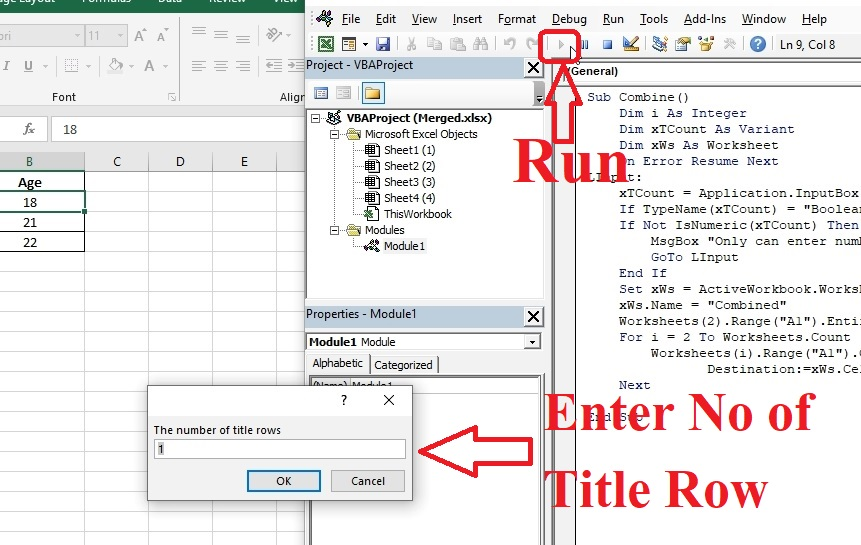5. Here is all sheets are combined in one sheet.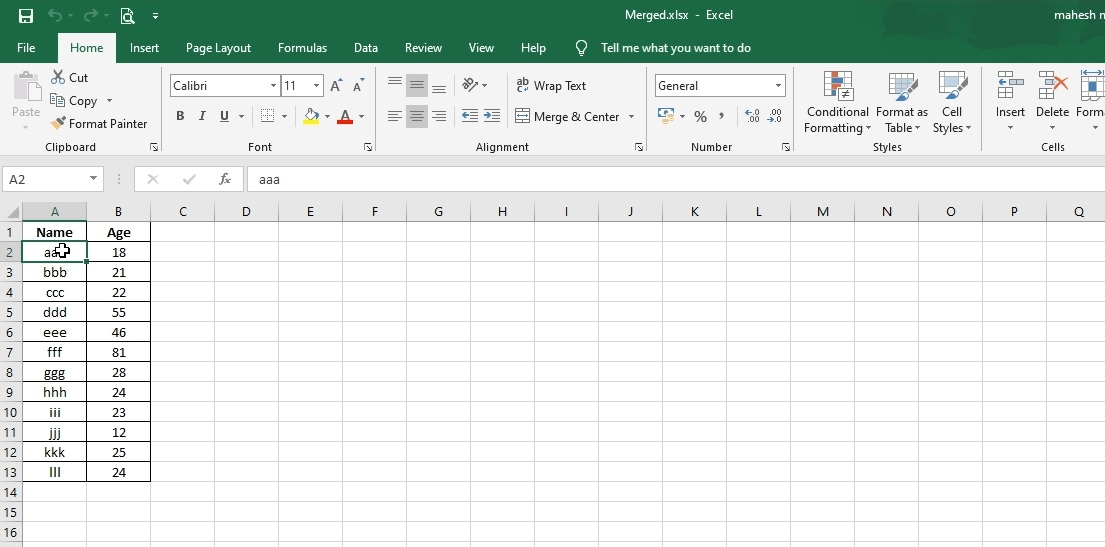Commnets 0